Author: Oscar Cronquist Article last updated on November 22, 2020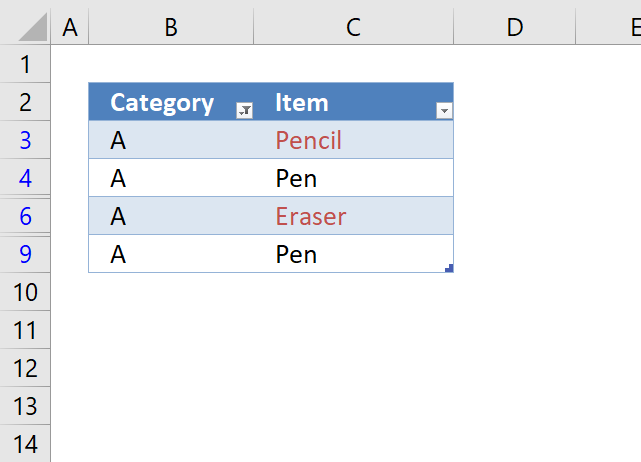This article demonstrates a conditional formatting formula that allows you to highlight unique values based on a set of filtered values using an Excel Table.

The image above shows an Excel Table that has a filter applied to column B or Table column header name "Category". A conditional formatting formula highlights unique values in cell range C3:C9.

Items "Pencil" and Eraser" are unique because they exist only once each in the filtered Excel Table, Item "Pen" exists in two different cells and are not highlighted.

Conditional Formatting has some amazing built-in features, for example, it lets you highlight unique values in a list without entering a CF formula. If you don't know how to do this using the built-in tool, follow these instructions:

1. Select your list.
2. Go to "Home" tab on the ribbon.
3. Press with left mouse button on the Conditional formatting button.
4. Hover over "Highlight Cells Rules".
5. Press with left mouse button on "Duplicate values...".
6. Change to "Unique".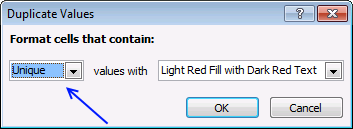7. Press with left mouse button on OK

However, if you then decide to filter the list based on a condition, the CF rule still highlights unique values as if it is not filtered.

The following CF formula highlights unique values in a filtered Excel Table.

=SUM(COUNTIF(INDIRECT("Table3[@Item]"), IF(SUBTOTAL(3, OFFSET(INDIRECT("Table3[Item]"), MATCH(ROW(INDIRECT("Table3[Item]")), ROW(INDIRECT("Table3[Item]")))-1, 0, 1)), INDIRECT("Table3[Item]"), "")))=1

The image below shows the filtered Excel table to the left and the same Excel Table to the right, however unfiltered, side by side. The Conditonal Formatting formula is applied to the Table to the left and the built-in tool is applied to the table to the right.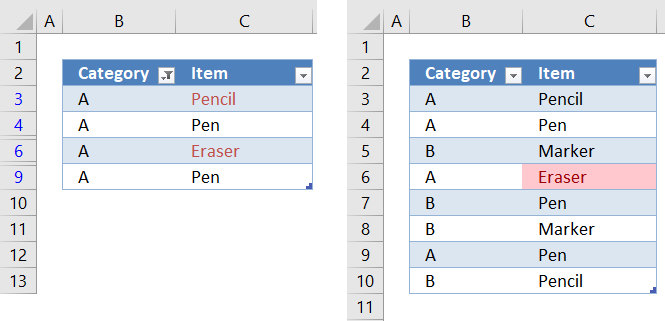The built-in tool is not able to correctly highlight unique values in a filtered Excel Table, shown below. Item "Pencil" should have been highlighted as well, it is unique in the filtered Excel Table. The Conditional formatting formula, however, is highlighting Item "Pencil" correctly in the Table to the left in the image above.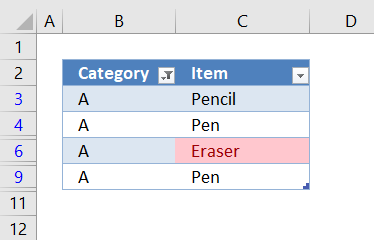### How to apply a CF formula rule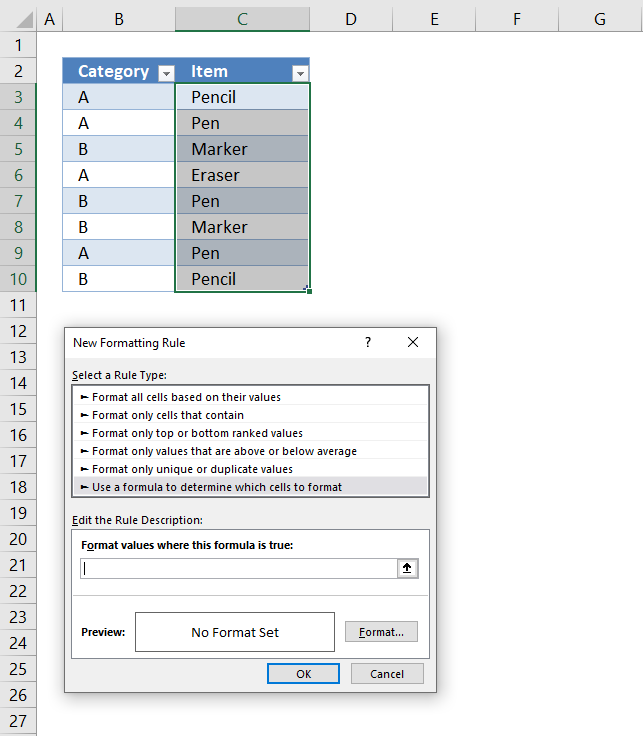You probably use a different table name on our worksheet, make sure you change the name accordingly in the CF formula.

1. Select the table column or list.
2. Go to "Home" tab on the ribbon.
3. Press with left mouse button on Conditional formatting button.
4. Press with left mouse button on "New rule...".
5. Press with left mouse button on "Use a formula to determine which cells to format".
6. Paste the above formula in this field.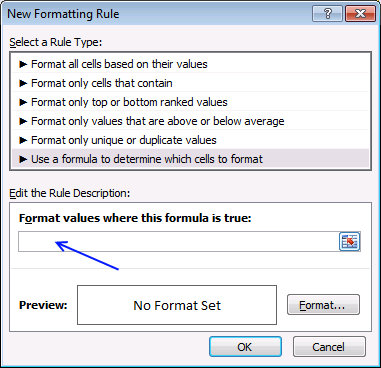7. Press with left mouse button on "Format..." button.
8. Go to tab "Fill".
9. Pick a background color.
10. Press with left mouse button on OK button twice.

### Explaining Conditional Formatting formula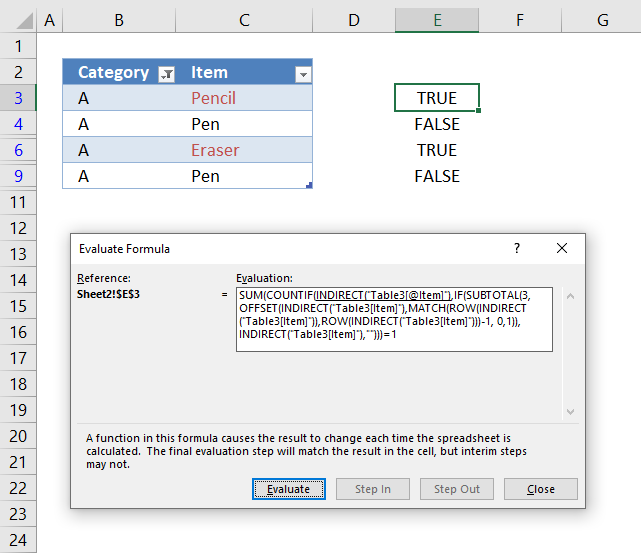I highly recommend the "Evaluate Formula" tool to understand and troubleshoot complicated formulas. It allows you to see calculation steps in greater detail at a pace you can control.

In order to use the "Evaluate Formula" tool you need to copy the CF formula and paste it to a cell next to the Excel Table. Copy the cell and paste to cells below as far as needed.

The image above shows the CF formula in cell E3 and cells below. The cell is highlighted if the CF formula evaluates to the boolean value TRUE.

Select a cell containing the formula you want to understand better, press with left mouse button on the "Formula" tab on the ribbon. Press with left mouse button on the "Evaluate Formula" button, a dialog box appears, see image above.

It shows the formula, the underlined expression is next to be evaluated. The lates evaluated expression is italicized. Press with left mouse button on the "Evaluate" button to see the next calculation step. Press with left mouse button on "Close" button to dismiss the dialog box.

#### Step 1 - Reference the value in the Excel Table on the same row as the Conditional formatting

Referencing a cell in an Excel Table is different than a regular cell, they are named structured references. First the table name then the column name inside brackets. The @ character tells you that the cell is in the same row as the formula.

Table3[@Item]

The Conditional Formatting formula is applied to cell C3 and Table3[@Item] references the same cell, however, it doesn't have to be the same cell. It can be a different cell but in the same row.

#### Step 2 - Enable Excel Table references in Conditional Formatting formulas

You can't use structured cell references in a CF formula, however, there is a workaround. Use the INDIRECT function, unfortunately, there is a downside with this approach which is necessary to keep in mind. If you change the name of the Excel Table the structured reference does not change automatically which otherwise would be the case.

INDIRECT("Table3[@Item]")

returns

"Pencil"

#### Step 3 - Enable arrays in the SUBTOTAL function

The SUBTOTAL function is able to identify if a cell is filtered or not but you can't use an array of values unless you apply this workaround. The OFFSET function is somehow capable of creating an array that you can use in the SUBTOTAL function.

OFFSET(INDIRECT("Table3[Item]"),MATCH(ROW(INDIRECT("Table3[Item]")),ROW(INDIRECT("Table3[Item]")))-1, 0,1))

becomes

OFFSET({"Pencil"; "Pen"; "Marker"; "Eraser"; "Pen"; "Marker"; "Pen"; "Pencil"},MATCH(ROW({"Pencil"; "Pen"; "Marker"; "Eraser"; "Pen"; "Marker"; "Pen"; "Pencil"}")),ROW({"Pencil"; "Pen"; "Marker"; "Eraser"; "Pen"; "Marker"; "Pen"; "Pencil"}))-1, 0,1))

becomes

OFFSET({"Pencil"; "Pen"; "Marker"; "Eraser"; "Pen"; "Marker"; "Pen"; "Pencil"},MATCH({3;4;5;6;7;8;9;10}),{3;4;5;6;7;8;9;10})-1, 0,1))

becomes

OFFSET({"Pencil"; "Pen"; "Marker"; "Eraser"; "Pen"; "Marker"; "Pen"; "Pencil"},{1;2;3;4;5;6;7;8}-1, 0,1))

becomes

OFFSET({"Pencil"; "Pen"; "Marker"; "Eraser"; "Pen"; "Marker"; "Pen"; "Pencil"},{1;2;3;4;5;6;7;8}-1, 0,1))

OFFSET({"Pencil"; "Pen"; "Marker"; "Eraser"; "Pen"; "Marker"; "Pen"; "Pencil"},{0;1;2;3;4;5;6;7}, 0,1))

and returns

{"Pencil";"Pen";"Marker";"Eraser";"Pen";"Marker";"Pen";"Pencil"}

The "Evaluate Formula" tool shows {#VALUE!; #VALUE!; #VALUE!; #VALUE!; #VALUE!; #VALUE!; #VALUE!; #VALUE!} but it still works.

#### Step 4 - Identify filtered values

SUBTOTAL(3,OFFSET(INDIRECT("Table3[Item]"),MATCH(ROW(INDIRECT("Table3[Item]")),ROW(INDIRECT("Table3[Item]")))-1, 0,1))

becomes

SUBTOTAL(3, {"Pencil";"Pen";"Marker";"Eraser";"Pen";"Marker";"Pen";"Pencil"})

and returns

{1; 1; 0; 1; 0; 0; 1; 0}

1 is visible, 0 (zero is hidden). The order of the values is important, they match the position of the values in column Item.

#### Step 5 - Replace the array with values

The IF function replaces 1 with the actual value corresponding to the position in the array and returns nothin "" if the value is 0 (zero)

IF(SUBTOTAL(3, OFFSET(INDIRECT("Table3[Item]"), MATCH(ROW(INDIRECT("Table3[Item]")), ROW(INDIRECT("Table3[Item]")))-1, 0, 1)), INDIRECT("Table3[Item]"), "")

becomes

IF({1; 1; 0; 1; 0; 0; 1; 0}, INDIRECT("Table3[Item]"), "")

becomes

IF({1; 1; 0; 1; 0; 0; 1; 0}, {"Pencil";"Pen";"Marker";"Eraser";"Pen";"Marker";"Pen";"Pencil"}, "")

and returns

{"Pencil";"Pen";"";"Eraser";"";"";"Pen";""}

These value are the ones that are visible if you filter column "Category" based on "A".#### Step 6 - Count values based on a condition

The COUNTIF function counts the visible values based on a condition which makes it possible to calculate if a value is unique or not.

COUNTIF(INDIRECT("Table3[@Item]"), IF(SUBTOTAL(3, OFFSET(INDIRECT("Table3[Item]"), MATCH(ROW(INDIRECT("Table3[Item]")), ROW(INDIRECT("Table3[Item]")))-1, 0, 1)), INDIRECT("Table3[Item]"), ""))

becomes

COUNTIF(INDIRECT("Table3[@Item]"), {"Pencil";"Pen";"";"Eraser";"";"";"Pen";""})

becomes

COUNTIF("Pencil", {"Pencil";"Pen";"";"Eraser";"";"";"Pen";""})

and returns

{1; 0; 0; 0; 0; 0; 0; 0}

#### Step 7 - Check if value is unique

The SUM function adds the numbers in the array and returns the total. We know that the value is unique if the total is equal to 1 meaning there is only one instance of that particular value.

SUM(COUNTIF(INDIRECT("Table3[@Item]"), IF(SUBTOTAL(3, OFFSET(INDIRECT("Table3[Item]"), MATCH(ROW(INDIRECT("Table3[Item]")), ROW(INDIRECT("Table3[Item]")))-1, 0, 1)), INDIRECT("Table3[Item]"), "")))=1

becomes

SUM({1; 0; 0; 0; 0; 0; 0; 0})=1

becomes

1=1

and returns True. Cell C3 is highlighted, see image below.If you want to learn more about advanced formulas check out my Advanced Excel Course. I have added two more videos, total 55 minutes. You can find them in the table of course contents. Expect more great videos in the near future.

### Get the Excel fileHighlight-unique-values-in-a-filtered-excel-tablev2.xlsx